Courses

# Magnetic Effects Of Current Practice Level - 1

## 25 Questions MCQ Test Exclusive Video Lectures of Class 12 Physics by Experts | Magnetic Effects Of Current Practice Level - 1

Description
This mock test of Magnetic Effects Of Current Practice Level - 1 for Class 12 helps you for every Class 12 entrance exam. This contains 25 Multiple Choice Questions for Class 12 Magnetic Effects Of Current Practice Level - 1 (mcq) to study with solutions a complete question bank. The solved questions answers in this Magnetic Effects Of Current Practice Level - 1 quiz give you a good mix of easy questions and tough questions. Class 12 students definitely take this Magnetic Effects Of Current Practice Level - 1 exercise for a better result in the exam. You can find other Magnetic Effects Of Current Practice Level - 1 extra questions, long questions & short questions for Class 12 on EduRev as well by searching above.
QUESTION: 1

Solution:
QUESTION: 2

Solution:
QUESTION: 3

### A coil having N turns is wound tightly in the form of aspiral with inner and outer radii a and b respectively. A current I passes through the coil. The magnetic moment of the spiral is

Solution:
QUESTION: 4

An a-particle moving in a circular orbit of radius a makes n revolutions per second. The magnetic field produced at the centre has a value B

Solution:
QUESTION: 5

A current carrying wire is placed in the grooves ofan insulating semi circular disc of radius 'R', asshown. The current enters at point A and leaves frompoint B. Determine the magnetic field at point D.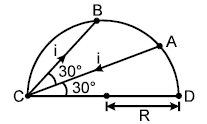Solution:
QUESTION: 6

A current I flows through a lengthy thin walled tube ofradius R having a longitudinal slit of width w. The magnetic field inside the tube at the axis under the condition w << R is

Solution:
QUESTION: 7

A long, straight metal rod has a long hole of radiusa drilled parallel to the rod axis with cross sectionalview shown below. If the rod carries a current I,the value of the magnetic field on the axis of therod is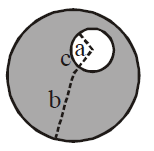Solution:
QUESTION: 8

Two circular coils A and B with their centres lying onthe same axis have same number of turns and carryequal currents in the same sense. They are separatedby a distance, have different diameters but subtendsame angle at a point P lying on their common axis.The coil B lies exactly midway between coil A andthe point P. The magnetic field at point P due to coils A and B is B1 and B2 respectively

Solution:
QUESTION: 9

Determine the magnetic field at the centre of thecurrent carrying wire arrangement shown in the figure.The arrangement extends to infinity. (The wires joiningthe successive squares are along the line passingthrough the centre)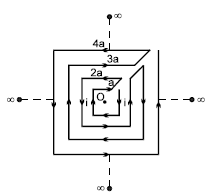Solution:
QUESTION: 10

A uniform surface charge density s exists on a sphere of radius a having a charge Q. The sphere is rotating with an angular velocity ω about its diameter. The equivalent magnetic moment is M.

Solution:
QUESTION: 11

A charged particle is released from rest in a region ofsteady and uniform electric and magnetic field whichare parallel to each other. The particle will move in a

Solution:
QUESTION: 12

Net magnetic field at the centre of the circle Odue to a current carrying loop as shown in figureis (q < 180°)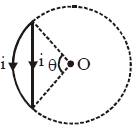Solution:
QUESTION: 13

In the figure shown ABCDEF A was a square loop of side l , but is folded in two equal parts so that half of itlies in x z plane and the other half lies in the y z plane.The origin ' O ' is centre of the frame also. The loopcarries current ' i '. The magnetic field at the centre is: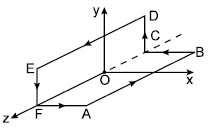Solution:
QUESTION: 14

Two identical particles having the same mass m andcharges +q and –q separated by a distance d enter ina uniform magnetic field B directed perpendicular topaper inwards with speeds v1 and v2 as shown infigure. The particles will not collide if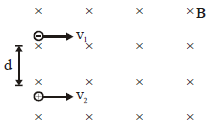Solution:
QUESTION: 15

A metallic wire is folded to form a square loop of sidea. It carries a current i and is kept perpendicular to auniform magnetic field. If the shape of the loop ischanged from square to a circle without changing thelength of the wire and current the amount of workdone in doing so is

Solution:
QUESTION: 16

Equal current i flows in two segments of a circularloop in the direction shown in the figure. Radius ofthe loop is a. Magnetic field at the centre of the loopisiq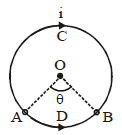Solution:
*Multiple options can be correct
QUESTION: 17

The magnetic field at the origin due to a current elementi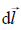placed at  a position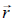is

Solution:
QUESTION: 18

A non conducting disc of radius R is rotating about an axis passing through its centre and perpendicular toits plane with an angular velocity ω. Charge q isuniformly distributed over its surface. The magnetic moment of the disc is

Solution:
QUESTION: 19

A rigid circular loop of radius r and mass m lies in thex-y plane on a flat table and has a current i flowing init. At this particular place, the earth’s magnetic field is B = Bxi + Bzk. The value of i so that one edge ofthe loop lifts from the table is

Solution:
QUESTION: 20

A large metal sheet carries an electric current alongits surface. Current per unit length is λ. Magnetic field near the metal sheet is

Solution:
*Multiple options can be correct
QUESTION: 21

A long, straight wire carries a current along the Z-axis.One can find two points in the X-Y plane such that

Solution:
QUESTION: 22

A cube made of wires of equal length is connected toa battery as shown in the figure. The side of cube is L. The magnetic field at the centre of the cube is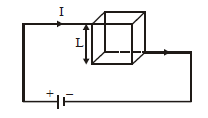Solution:
QUESTION: 23

A conducting rod of length l and mass m is moving down a smooth inclined plane of inclination Q with constant velocity v. A current i is flowing in the conductor in a direction perpendicular to paper inwards. A vertically upward magnetic field B exists in space. Then magnitude of magnetic field B is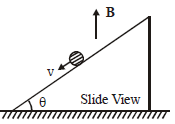Solution:
QUESTION: 24

In the figure shown a coil of single turn is wound on asphere of radius R and mass m. The plane of the coilis parallel to the plane and lies in the equatorial planeof the sphere. Current in the coil is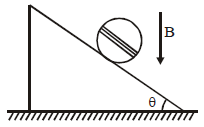Solution:
QUESTION: 25

A current carrying wire AB of the length 2πR is turnedalong a circle, as shown in figure. The magnetic field atthe centre O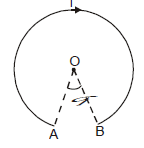Solution: